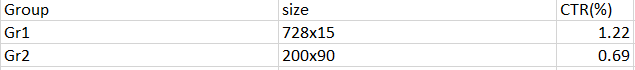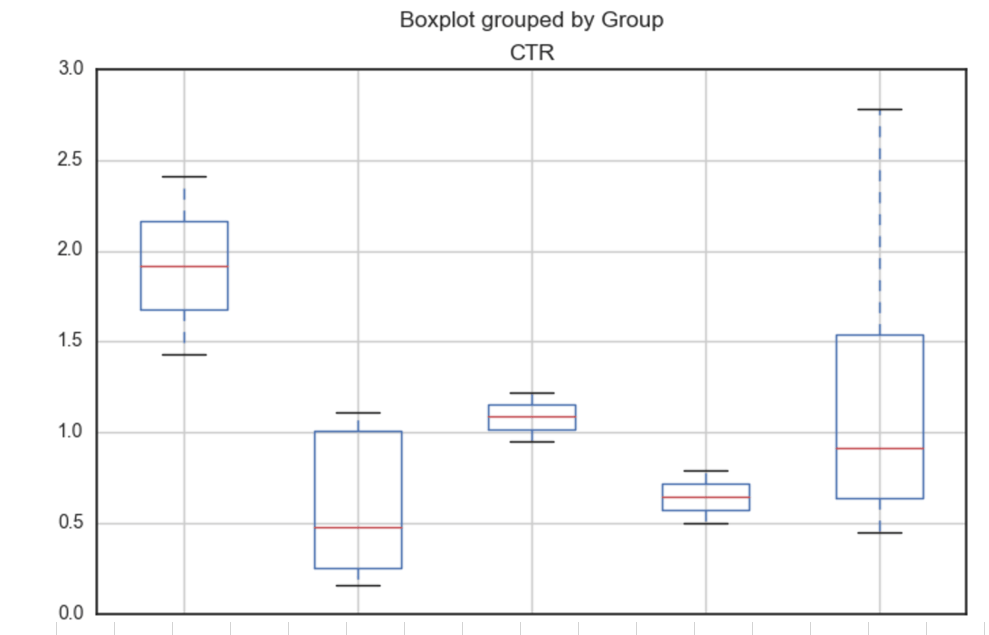## Exploratory Data Analysis (EDA) – No Programming is Needed

In statistics, exploratory data analysis (EDA) is an approach to analyzing data sets to summarize their main characteristics, often with visual methods. A statistical model can be used or not, but primarily EDA is for seeing what the data can tell us beyond the formal modeling or hypothesis testing task. 

According to  Box plot is one of typical graphical techniques used in EDA.

A box plot or boxplot is a convenient way of graphically depicting groups of numerical data through their quartiles. Box plots may also have lines extending vertically from the boxes (whiskers) indicating variability outside the upper and lower quartiles, hence the terms box-and-whisker plot and box-and-whisker diagram. Outliers may be plotted as individual points. 

In this post we will consider how to create box plot for EDA of data from website without using programming. We will use online tool ML Sandbox from this site and the data from Google AdSense and Google Analytics. Here is the sample of few rows:To use the tool we need select “Exploratory Data Analysis” in menu options and then enter data into Input Data Exploratory Data Analysis text field.
Please note that the data should have header field as the first row. Also it is important that the first column should be class column or the column that you use in group by. This field will be on X axis of box plot. It can be text data field. The other columns should be numerical, they will be on Y axis of box plot. In our case we enter data 2 times, with data columns as below:
1. Group, CTR(%) Columns
2. Size, CTR(%) Columns

Each time, after we enter data we click Run Now and then click results link. We might need wait a little and click Refresh button few times untill data results show up.

Here are screenshots of boxplots. We see how the data are distributed for different groups (classes) based on the five number summary: minimum, first quartile, median, third quartile, and maximum.Box plots are useful for identifying outliers and for comparing distributions. Do you want to get the insights into your data? Then visit ML Sandbox and use EDA option to build box plot.

References
1. Exploratory data analysis Wikipedia
2. Box plot Wikipedia
3. ML Sandbox
4. Box Plot: Display of Distribution